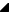﻿ TRSLocalChartAxis Methods# TRSLocalChartAxis MethodsMethods

Name

DescriptionAxisToPixel(TRSChartValueType)

Converts from the chart value parameter to a pixel coordinate of the Owner RSChartPanel.TRSCustomChartPanel component. Based on the     ZoomMinimum and     ZoomMaximum range of the chart axis, this routine converts the parameter value into a pixel coordinate. Unlike the overloaded AxisToPixel method, this method assumes that the visual pixel range of the chart axis is defined by the chart panel's     ChartRect property. The chart axis uses the bounds of the rectangle that apply to its location, e.g., a BottomAxis chart axis would use the ChartRect's Left=ZoomMinimum and Right=ZoomMaximum fields for the calculation (the rectangle is also adjusted if StartPosition and EndPosition properties are not their defaults).

Use one of the AxisToPixel methods anytime you need to convert from internal chart axis coordinates to a visual pixel coordinate. For example, if the chart axis has a zoomed range from 0 to 100 and the ChartRect is Left=150 and Right=500, this function would return:
AxisToPixel( 0 ) = 150
AxisToPixel( 50 ) = 325
AxisToPixel( 100 ) = 500

Use one of the     PixelToAxis methods to convert back from pixel coordinates to chart coordinates.PixelToAxis(TCanvasPixel,Boolean)

Converts from a canvas pixel coordinate to a chart axis coordinate. Based on the     ZoomMinimum and     ZoomMaximum range of the chart axis, this routine converts the parameter value into a chart axis coordinate. Unlike the overloaded PixelToAxis method, this method assumes that the visual pixel range of the chart axis is defined by the chart panel's ChartRect property (obtained through the Owner property). The chart axis uses the bounds of the rectangle that apply to its location, e.g., a BottomAxis chart axis would use the ChartRect's Left=ZoomMinimum and Right=ZoomMaximum fields for the calculation (the rectangle is also adjusted if StartPosition and EndPosition properties are not their defaults).

Use one of the PixelToAxis methods anytime you need to convert from a canvas pixel coordinate to the internal chart axis coordinates. For example, if the chart axis has a zoomed range from 0 to 100 and the ChartRect is Left=150 and Right=500, this function would return:
PixelToAxis( 150 ) = 0
PixelToAxis( 325 ) = 50
PixelToAxis( 500 ) = 100

Use one of the AxisToPixel methods to convert back from chart coordinates to canvas pixel coordinates.

Top

RiverSoftAVG Charting Component Suite (RCCS) © 2005-2015, Thomas G. Grubb